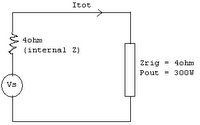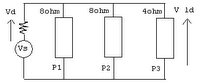## Pages

### Power handling pt.3

In this post I will recapitulate what we have learned from the previous two posts. I will use my rig as an example:

- Behringer 3000T 300W @4ohms Bass Head
- Behringer BA210 500W 8ohm Cabinet (2 x 10")
- Behringer BA115 600W 8ohm Cabinet (1 x 15")

First things first...We need to calculate the maximum distortion-free output voltage of the amplifier, Vs. This is a constant parameter which depends on the amplifier characteristics (output power and internal impedance). From the above info, we can say that if we connect a 4ohm load to the amplifier (match impedance with impedance of amplifier). the delivered power (power on the load) will be 300W:P out = (I tot)^2 * (Z rig)
300 = (I tot)^2 * 4
=> I tot = 8.66A

But, Required Power (power driven from amplifier) can be found from:
P req = (I tot)^2 * (Z tot)
= (8.66)^2 * (4 + 4)
=> P req = 600W

Referring to the above diagram, the maximum distortion free output voltage can be found from:

Vs = (P req) / I tot
= 600 / 8.66

=> Vs = 69.3V

Now that we have calculated the maximum output voltage of the amplifier, we can solve the puzzle:

How much power is delivered/required if I connect both the Behringer cabs?
With both Behringer cabs connected in parallel, we will have a total impedance of 4ohms. We will basically end up with the same circuit in the first diagram. Therefore we know that total current is:

I tot = 8.66A

Therefore P out = (8.66)^2 * 4
=> P out = 300W

and, P req = (8.66) ^ 2 * (4 + 4)
=> P req = 600W

How much power is delivered/required if I connect only one of the Behringer cabs?
With just one cab connected to the amplifier, I will have an 8ohm load across the amplifier. Using the same equations together with the popular V = I * R:

I tot = Vs / Z tot
= 69.3 / (4 + 8)
= 5.77A

Therefore P out = (5.77)^2 * 8
=> P out = 267W

and, P req = (5.77) ^ 2 * (4 + 8)
=> P req = 400W

How much power is delivered/required if I connect a 4ohm cab to my rig?
We are now presenting a load total of 2ohm to the amplifier. More current will flow compared to the previous scenario, so we are driving the amp harder, risking to blow it up. Using the same equations once again:

I tot = Vs / Z tot
= 69.3 / (4 + 2)
= 11.55A

Therefore P out = (11.55)^2 * 2
=> P out = 267W

and, P req = (11.55) ^ 2 * (4 + 2)
=> P req = 800W

Now comes the final question. Let's depict it by using a complex scenario. Assume that my 2ohm rig is connected as in the below diagram.Will each cab be able to handle the power being presented to it, assuming that the cabs have a maximum handling power of 500W each?
From the previous step, I tot = 11.55A. This means that the voltage drop in the amplifier's internal resistance is:

Vd = I tot * Internal Resistance
Vd = 11.55 * 4
Vd = 46.2V

Thus, the voltage across rig,

V ld = Vs - Vd
V ld = 69.3 - 46.2
V ld = 23.1V

Therefore, using the formula Power = V^2 / R:

P1 = 23.1^2 / 8 = 66.7W
P2 = 23.1^2 / 8 = 66.7W
P3 = 23.1^2 / 4 = 133.4W

This means that all of the cabs will handle the power delivered to them.
Note that if you sum all the power delivered per speaker, you will obtain the total delivered power. This shows that power is an additive quantity, whether the load is connected in series or in parallel. In layman's words, if I have a 300W sound system with two similar speakers (in terms of impedance), I will have 150W delivered from each speaker.